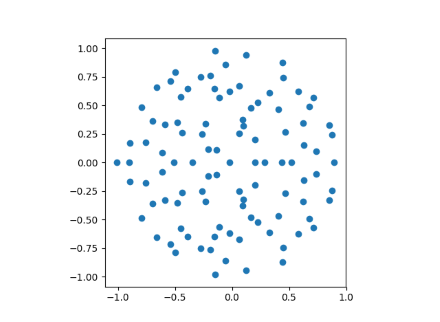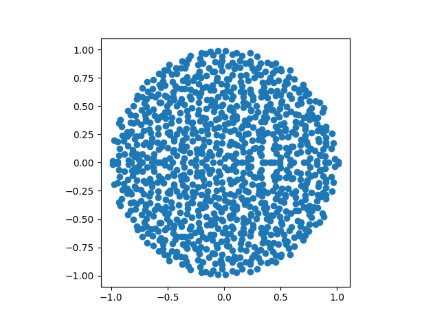{{announcement.body}}
{{announcement.title}}

# Girko-Ginibri Circular Law for Random Matrix Eigenvalues

DZone 's Guide to

# Girko-Ginibri Circular Law for Random Matrix Eigenvalues

### A math whiz and programming expert looks at how to work with random data sets using the Girko-Ginibri Circular Law, and implements it in Python.

· Big Data Zone ·
Free Resource

Comment (0)

Save
{{ articles.views | formatCount}} Views

Suppose you create a large matrix by filling its components with random values. If M has size n by n, then we require the probability distribution for each entry to have mean 0 and variance 1/n. Then the Girko-Ginibri circular law says that the eigenvalues of M are approximately uniformly distributed in the unit disk in the complex plane. As the size n increases, the distribution converges to a uniform distribution on the unit disk.

The probability distribution need not be normal. It can be any distribution, shifted to have mean 0 and scaled to have variance 1/n, provided the tail of the distribution isn't so thick that the variance doesn't exist. If you don't scale the variance to 1/n you still get a circle, just not a unit circle.

We'll illustrate the circular law with a uniform distribution. The uniform distribution has mean 1/2 and variance 1/12, so we will subtract 1/2 and multiply each entry by √(12/n).

Here's our Python code:

``````    import matplotlib.pyplot as plt
import numpy as np

n = 100
M = np.random.random((n,n)) - 0.5
M *= (12/n)**0.5
w = np.linalg.eigvals(M)
plt.scatter(np.real(w), np.imag(w))
plt.axes().set_aspect(1)
plt.show()``````

When n=100 we get the following plot.When n=1000 we can see the disk filling in more.Note that the points are symmetric about the real axis. All the entries of M are real, so its characteristic polynomial has all real coefficients, and so its roots come in conjugate pairs. If we randomly generated complex entries for M we would not have such symmetry.

Topics:
big data ,data visualization ,python ,random data ,tutorial

Comment (0)

Save
{{ articles.views | formatCount}} Views

Published at DZone with permission of John Cook , DZone MVB. See the original article here.

Opinions expressed by DZone contributors are their own.